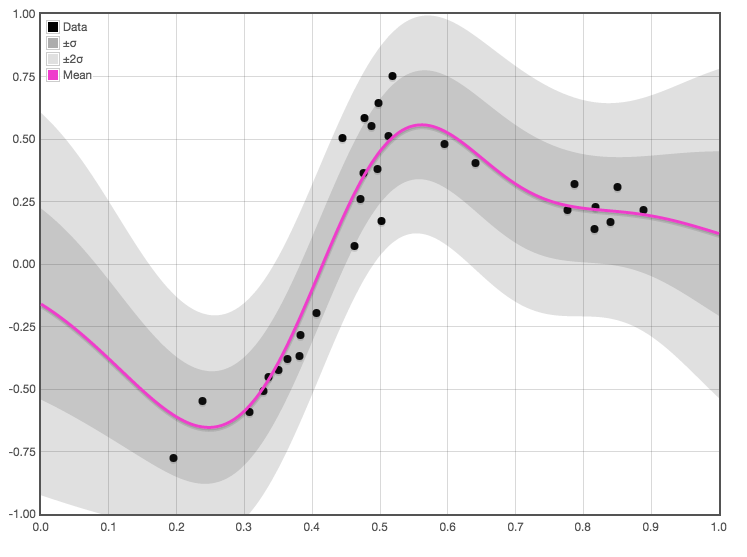# Gaussian process regression

## And classification. And extensions.Gaussian random processes/fields are stochastic processes/fields with jointly Gaussian distributions of observations. While “Gaussian process regression” is not wrong per se, there is a common convention in stochastic process theory (and also in pedagogy) to use process to talk about some notionally time-indexed process and field to talk about ones that have a some space-like index without a presumption of an arrow of time. This leads to much confusion, because Gaussian field regression is what we usually want to talk about. What we want to use the arrow of time for is a whole other story. Regardless, hereafter I’ll use “field” and “process” interchangeably.

In machine learning, Gaussian fields are used often as a means of regression or classification, since it is fairly easy to conditionalize a Gaussian field on data and produce a posterior distribution over functions. They provide nonparametric method of inferring regression functions, with a conveniently Bayesian interpretation and reasonably elegant learning and inference steps. I would further add that this is the crystal meth of machine learning methods, in terms of the addictiveness, and of the passion of the people who use it.

The central trick is using a clever union of Hilbert space trickss and probability to give a probabilistic interpretation of functional regression as a kind of nonparametric Bayesian inference.

Useful side divergence into representer theorems and Karhunen-Loève expansions for thinking about this. Regression using Gaussian processes is common e.g. spatial statistics where it arises as kriging. Cressie (1990) traces a history of this idea via Matheron (1963a), to works of Krige (1951).This web site aims to provide an overview of resources concerned with probabilistic modeling, inference and learning based on Gaussian processes. Although Gaussian processes have a long history in the field of statistics, they seem to have been employed extensively only in niche areas. With the advent of kernel machines in the machine learning community, models based on Gaussian processes have become commonplace for problems of regression (kriging) and classification as well as a host of more specialized applications.

I’ve not been enthusiastic about these in the past. It’s nice to have a principled nonparametric Bayesian formalism, but it has always seemed pointless having a formalism that is so computationally demanding that people don’t try to use more than a thousand data points, or spend most of a paper working out how to approximate this simple elegant model with a complex messy model. However, that previous sentence describes most of my career now, so I guess I must have come around.

Perhaps I should be persuaded by tricks such as AutoGP which breaks some computational deadlocks by clever use of inducing variables and variational approximation to produce a compressed representation of the data with tractable inference and model selection, including kernel selection, and doing the whole thing in many dimensions simultaneously. There are other clever tricks like this one, e.g shows how to use a lattice structure for observations to make computation cheap.

## Quick intro

I am not the right guy to provide the canonical introduction, because it already exists. Specifically, Rasmussen and Williams (2006).

This lecture by the late David Mackay is probably good; the man could talk.

There is also a well-illustrated and elementary introduction by Yuge Shi. There are many, many more.

J. T. Wilson et al. (2021):

A Gaussian process (GP) is a random function $$f: \mathcal{X} \rightarrow \mathbb{R}$$, such that, for any finite collection of points $$\mathbf{X} \subset \mathcal{X}$$, the random vector $$\boldsymbol{f}=f(\mathbf{X})$$ follows a Gaussian distribution. Such a process is uniquely identified by a mean function $$\mu: \mathcal{X} \rightarrow \mathbb{R}$$ and a positive semi-definite kernel $$k: \mathcal{X} \times \mathcal{X} \rightarrow \mathbb{R}$$. Hence, if $$f \sim \mathcal{G} \mathcal{P}(\mu, k)$$, then $$\boldsymbol{f} \sim \mathcal{N}(\boldsymbol{\mu}, \mathbf{K})$$ is multivariate normal with mean $$\boldsymbol{\mu}=\mu(\mathbf{X})$$ and covariance $$\mathbf{K}=k(\mathbf{X}, \mathbf{X})$$.

[…] we investigate different ways of reasoning about the random variable $$\boldsymbol{f}_* \mid \boldsymbol{f}_n=\boldsymbol{y}$$ for some non-trivial partition $$\boldsymbol{f}=\boldsymbol{f}_n \oplus \boldsymbol{f}_*$$. Here, $$\boldsymbol{f}_n=f\left(\mathbf{X}_n\right)$$ are process values at a set of training locations $$\mathbf{X}_n \subset \mathbf{X}$$ where we would like to introduce a condition $$\boldsymbol{f}_n=\boldsymbol{y}$$, while $$\boldsymbol{f}_*=f\left(\mathbf{X}_*\right)$$ are process values at a set of test locations $$\mathbf{X}_* \subset \mathbf{X}$$ where we would like to obtain a random variable $$\boldsymbol{f}_* \mid \boldsymbol{f}_n=\boldsymbol{y}$$.

[…] we may obtain $$\boldsymbol{f}_* \mid \boldsymbol{y}$$ by first finding its conditional distribution. Since process values $$\left(\boldsymbol{f}_n, \boldsymbol{f}_*\right)$$ are defined as jointly Gaussian, this procedure closely resembles that of [the finite-dimensinal case]: we factor out the marginal distribution of $$\boldsymbol{f}_n$$ from the joint distribution $$p\left(\boldsymbol{f}_n, \boldsymbol{f}_*\right)$$ and, upon canceling, identify the remaining distribution as $$p\left(\boldsymbol{f}_* \mid \boldsymbol{y}\right)$$. Having done so, we find that the conditional distribution is the Gaussian $$\mathcal{N}\left(\boldsymbol{\mu}_{* \mid y}, \mathbf{K}_{*, * \mid y}\right)$$ with moments \begin{aligned} \boldsymbol{\mu}_{* \mid \boldsymbol{y}}&=\boldsymbol{\mu}_*+\mathbf{K}_{*, n} \mathbf{K}_{n, n}^{-1}\left(\boldsymbol{y}-\boldsymbol{\mu}_n\right) \\ \mathbf{K}_{*, * \mid \boldsymbol{y}}&=\mathbf{K}_{*, *}-\mathbf{K}_{*, n} \mathbf{K}_{n, n}^{-1} \mathbf{K}_{n, *}\end{aligned}

## Observation likelihoods

Classification etc. TBD

## Incorporating a mean function

Almost immediate but not quite trivial .

TODO: discuss identifiability.

## Density estimation

Can I infer a density using GPs? Yes. One popular method is apparently the logistic Gaussian process.

## Kernels

a.k.a. covariance models.

GP regression models are kernel machines. As such covariance kernels are the parameters. More or less. One can also parameterise with a mean function, but let us ignore that detail for now because usually we do not use them.

## Using state filtering

When one dimension of the input vector can be interpreted as a time dimension we are Kalman filtering Gaussian Processes, which has benefits in terms of speed.

## On manifolds

I would like to read Terenin on GPs on Manifolds who also makes a suggestive connection to SDEs, which is the filtering GPs trick again.

🏗

## Neural processes

See neural processes.

## With inducing variables

“Sparse GP”. See Quiñonero-Candela and Rasmussen (2005). 🏗

## By variational inference with inducing variables

See GP factoring.

## Approximation with dropout

See NN ensembles.

## Inhomogeneous with covariates

Integrated nested Laplace approximation connects to GP-as-SDE idea, I think?

e.g. GP-LVM . 🏗